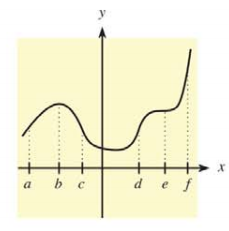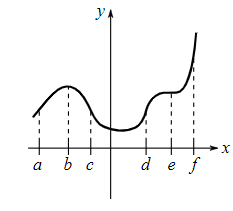Chapter 10.2, Problem 5E### Mathematical Applications for the ...

11th Edition
Ronald J. Harshbarger + 1 other
ISBN: 9781305108042

#### Solutions

Chapter
Section### Mathematical Applications for the ...

11th Edition
Ronald J. Harshbarger + 1 other
ISBN: 9781305108042
Textbook Problem

# In Problems 5-10, use the indicated x-values on the graph of y = f ( x ) to find the following.Find intervals where f " ( x ) > 0.

To determine

The intervals over which f(x)>0Explanation

Given Information:

The provided graph is,

Explanation:

Consider the provided graph,

The slope of function f(x) is increasing in the interval (c,d) and second derivative f(x) of function f(x) is positive in the interval (c,d) that is f(x)>0

### Still sussing out bartleby?

Check out a sample textbook solution.

See a sample solution

#### The Solution to Your Study Problems

Bartleby provides explanations to thousands of textbook problems written by our experts, many with advanced degrees!

Get Started

#### Find more solutions based on key concepts